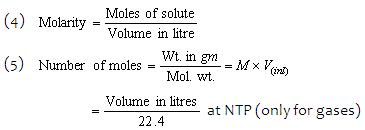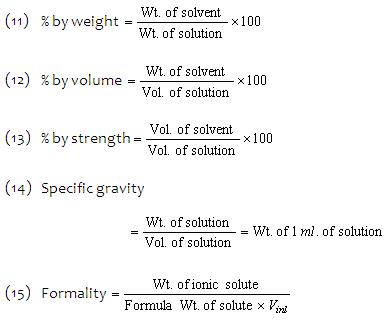1800-1023-196

+91-120-4616500

# Formula Used in Volumetric Analysis

## Formula Used in Volumetric Analysis

Formula used in solving numerical problems on volumetric analysis:

(1) Strength of solution = Amount of substance in g litre-1

(2) Strength of solution = Amount of substance in g moles litre-1

(3) Strength of solution = Normality × Eq. wt. of the solute

=  molarity × Mol. wt. of solute(6) Number of millimoles = Wt. in gm × 1000/mol.wt.
= Molarity × Volume in Ml.
(7) Number of equivalents
=  Wt. in (gm/Eq. wt.) = x × No. of mole × Normality × Volume in litre
(8) Number of milliequivalents (meq.)
= (Wt. in gm × 1000/Eq.wt.) = normality × Volume in ml.
(9) Normality = x× No. of millimoles
= x× Molarity = (Strength in gm litre-1/Eq.wt.)
where x = (Mol. Wt./Eq.wt.), x = valency or change in oxi. Number.
(10) Normality formula, N1V1 = N2V2(16) Mol. Wt. = V.D × 2 (For gases only)

## NEET & AIIMS Exam Sample Papers

 AIIMS SAMPLE PAPERS View More NEET SAMPLE PAPERS View More